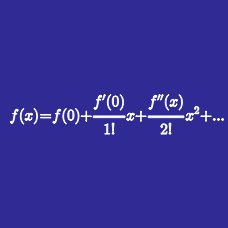Calculus

# Taylor Series Approximation

Let $f(x)$ be a function such that $f(0)=3, f'(0)=1,$ and $f(3)=1.$ Using a quadratic Taylor polynomial of $f(x),$ we can approximate the value of $f''(0)$ as $f''(0) \approx -\frac{A}{B},$ where $A$ and $B$ are coprime integers. Find the value of $AB.$

Using the quadratic Taylor polynomial of $f(x) = \frac{1}{x}$ at $x=5,$ find the approximate value of $\frac{1}{7}$ multiplied by $5^3.$

For the linear Taylor polynomial $g(x)=ax+b$ of $f(x)=-\frac{1}{x-1}$ at $x=2,$ find the error $e$ defined by $e=\int_{2}^{3}{(g(x)-f(x))^2 dx}.$

Using the quadratic Taylor polynomial of $f(x) = \ln{x}$ at $x=4,$ we can approximate the value of $\ln{9}$ in the form $\ln{A}+B,$ where $A$ and $B$ are rational numbers. Find the value of $2A^2B.$

Let $f(x)$ be a function such that $f(0)=1, f'(0)=2,$ and $f(3)=1.$ Using a quadratic Taylor polynomial of $f(x),$ we can approximate the value of $f''(0)$ as $f''(0) \approx -\frac{A}{B},$ where $A$ and $B$ are coprime integers. Find the value of $AB.$

×